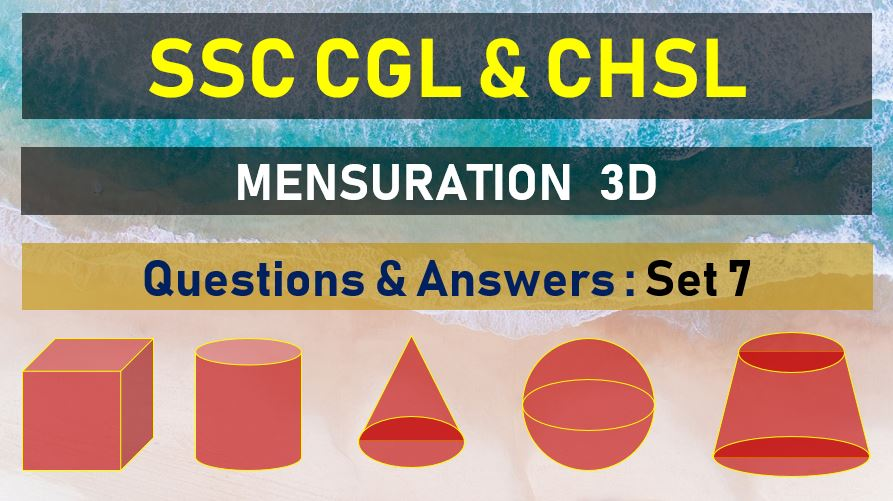Tuesday, June 22, 2021
Home > mensuration > SSC CGL Mensuration 3D Questions Answers Set 7

# SSC CGL Mensuration 3D Questions Answers Set 7SSC Mensurations 3D
Surface Area & Volume Questions Sets
Set 1Set 2Set 3Set 4Set 5
Set 6Set 7Set 8Set 9Set 10

SSC CGL Mensuration 3D Questions Answers Set 7: Ques No 1

The base of a solid right prism is a triangle whose sides are 9 cm, 12 cm and 15 cm, The height of the prism is 5 cm. Then the total surface area of the prism is

Options:

A. 180 cm2

B. 234 cm2

C. 288 cm2

D. 270 cm2

Answer: B

Solution:

SSC CGL Mensuration 3D Questions Answers Set 7: Ques No 2

The perimeter of the triangular base of a right prism is 15 cm and radius of the incircle of the triangular base is 3 cm. If the volume of the prism be 270 cm3 then the height of the prism is

Options:

A. 6 cm

B. 7.5 cm

C. 10 cm

D. 11 cm

Answer: D

Solution:

SSC CGL Mensuration 3D Questions Answers Set 7: Ques No 3

The base of a right prism is a trapezium. The length of the parallel sides are 8 cm and 14 cm and the distance between the parallel sides is 8 cm, if the volume of the prism is 1056 cm3, then the height of the prism is

Options:

A. 44 cm

B. 16.5 cm

C. 12 cm

D. 10.56 cm

Answer: C

Solution:

SSC CGL Mensuration 3D Questions Answers Set 7: Ques No 4

The base of a right pyramid is a surface of the prism is square of side 40 cm long. If the volume of the pyramid is 8000 cm3, then its height is

Options:

A. 5 cm

B. 10 cm

C. 15 cm

D. 20 cm

Answer: C

Solution:

SSC CGL Mensuration 3D Questions Answers Set 7: Ques No 5

There is a pyramid on a base which is a regular hexagon of Side 2a cm. if every slant edge of this pyramid is of length 5a/2 cm then the value of the pyramid is

Options:

A. 3a3 cm3

B. 3√2a3 cm3

C. 3√3a3 cm3

D. 6a3 cm3

Answer: C

Solution:

SSC CGL Mensuration 3D Questions Answer Set 7: Ques No 6

A right triangle with its sides 9 cm, 12 cm and 15 cm is revolved about the side 12 cm. Find the volume of the solid so formed.

Options:

A. 1018.28 cm3

B. 702.57 cm3

C. 1200 cm3

D. 1218.28 cm3

Answer: A

Solution:

SSC CGL Mensuration 3D Questions Answer Set 7: Ques No 7

If the radii of the circular ends of a truncated conical bucket which is 45cm high be 28 cm and 7cm then the capacity of the bucket in cubic centimeter is

Options:

A. 48510

B. 45810

C. 48150

D. 48051

Answer: A

Solution:

SSC CGL Mensuration 3D Questions Answer Set 7: Ques No 8

If the length of each side of a regular tetrahedron is 12 cm, then the volume of the tetrahedron is

Options:

A. 144√2 cu. Cm

B. 72√2 cu. Cm

C. 8√2 cu. Cm

D. 12√2 cu. Cm

Answer: A

Solution:

SSC CGL Mensuration 3D Questions Answer Set 7: Ques No 9

The shape of a solid is a cylinder surmounted by a cone. If the volume of the solid is 40656 cm3, the diameter of the base is 42 cm and the height of the cylinder is 20 cm, find the slant height of the conical portion.

Options:

A. 45 cm

B. 35 cm

C. 40 cm

D. 50 cm

Answer: B

Solution:

SSC CGL Mensuration 3D Questions Answer Set 7: Ques No 10

If the radius of a sphere be doubled, then the percentage increase in volume is

Options:

A. 500 %

B. 600 %

C. 700 %

D. 800 %

Answer: B

Solution:

SSC Mensurations 3D
Surface Area & Volume Questions Sets
Set 1Set 2Set 3Set 4Set 5
Set 6Set 7Set 8Set 9Set 10
error: Content is protected !!